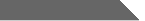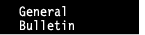About MiamiAcademicsAdmissionLiving at MiamiUniversity OfficesGeneral Bulletin 2008-2010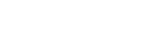Courses of Instruction

MATHEMATICS (MTH-Arts and Science; Department of Mathematics and Statistics)

Note:

1. Service courses do not count toward majors in the Department of Mathematics and Statistics. They may or may not count toward majors in other departments. Look carefully at your major requirements and at the mathematics and statistics placement guide in this Bulletin.
2. On regional campuses, placement into MTH 101, 102, 115, 125, and 151 is based on achieving an appropriate score on a standard placement test administered at the regional campus.

### 101 Introduction to Elementary Algebra (3)

Service course. Introduction to fundamental topics of beginning algebra. Primarily for students with no previous course in algebra. Offered only on Hamilton and Middletown campuses. Credit/no-credit only. Prerequisite: passing grade in MTH 002 or qualifying placement score.

### 102 Intermediate Algebra (3)

Service course. Introduction to functions and a study of algebra topics including radicals, quadratics, and rational expressions. Prerequisite: Passing grade in MTH 101 or qualifying placement score.

### 104 Precalculus with Algebra (5)

Service course. Functions, rational functions, logarithmic and exponential functions, trigonometry, along with review of algebra topics important for calculus. Prerequisite: two years of college preparatory mathematics, but little or no trigonometry.

Note: Credit for graduation will not be given for more than one of MTH 102 and 104 nor for more than one of MTH 104, 123, and 125. At most nine credit hours toward graduation can be earned from any combination of MTH 101, 102, 104, 123, and 125.

### MPF 115 Mathematics for Teachers of Grades P-6 (4)

Service course. Topics include problem solving, numeration, computation, number theory, and rational numbers. Designed to provide content background for teaching mathematics in elementary grades. Successful completion of this course may require an examination in basic mathematics. Open only to early childhood or middle childhood majors not concentrating in mathematics and special education majors. Prerequisite: two years of high school algebra or a college algebra course. V.

### 116 Mathematics for Elementary Teachers (4)

Service course. Topics are from geometry, probability, and statistics. Designed to provide content background for teaching mathematics in elementary grades. Open only to early childhood and special education majors.

### MPF 121 Finite Mathematical Models (3)

Service course. Introduction to linear, probabilistic, graph-theoretic, and network models with emphasis on development of algorithms. Systems of linear equations, linear programming, matrix algebra, graphs, networks, discrete probabilistic models, and linear recurrence relations with applications of these topics to areas in the management, social, and biological science. Prerequisite: MTH 102 or 104 or three years of college preparatory mathematics including Algebra II. V. CAS-E.

### 123 Precalculus (3)

Service course. Covers many topics important for calculus: functions, rational functions, logarithmic and exponential functions, trigonometry, and some analytic geometry. Prerequisite: three years of college preparatory mathematics including some trigonometry.

### 125 Precalculus (5)

Service course. Review of algebra topics important for calculus. Functions, polynomials, rational functions, logarithmic and exponential functions, trigonometric functions and their inverses, conic sections, nonlinear systems, and applications of functions. Offered only on Hamilton and Middletown campuses. Credit does not count toward a major in mathematics and statistics. Prerequisite: "C" or better in MTH 102 or qualifying placement score.

### 129 Precalculus Enrichment Workshop (2)

Service course. Content parallels MTH 104 and 123. Designed to enhance a regular precalculus offering, this course consists primarily of group work on projects of an extended character. Prerequisite: concurrent enrollment in MTH 104 or 123 and permission of department. Offered infrequently.

Note: Credit for graduation will not be given for more than one of MTH 151 or 153.

### MPF, MPT 151 Calculus I (5)

Limits and continuity, derivatives, integration, calculus of trigonometric and exponential functions. Prerequisite: three and a half or four years of college preparatory mathematics including trigonometry, but less than one semester of calculus. Or, one of MTH 104 or 123 or 125. V. CAS-E.

### MPF, MPT 153 Calculus I (4)

Covers same content as MTH 151, but assumes some previous study of calculus. (See MTH 151.) Prerequisite: four years of college preparatory mathematics including trigonometry and at least one semester of high school calculus. V. CAS-E.

### 157H Honors Calculus I (5)

Rigorous in-depth treatment of ideas found in Calculus I. Prerequisite: strong background in precalculus mathematics and permission of department.

### 159 Calculus Enrichment Workshop (2)

Content parallels calculus I ( MTH 151/153). Designed to enhance a regular calculus offering, this course consists primarily of group work on projects of an extended character. Prerequisite: concurrent enrollment in a calculus I course and permission of department.

### 175 Environmental Science Seminar (1)

Introduces the multidisciplinary nature of environmental science and the solution of environmental problems. This course does not meet any CAS requirements. Cross-listed with BOT/CHM/GEO/GLG/MBI/STA/ ZOO 175

### 190 First Year Seminar in Mathematics and Statistics (1; maximum 1)

Seminar groups explore and discuss topics in mathematics and statistics. Credit/no credit only. Prerequisite: completion of or concurrent enrollment in Calculus I (or above) or permission of instructor.

### 217 Mathematics for Middle Childhood Teachers: Structure of Arithmetic and Algebra (3)

Service Course. A systematic study of the underlying properties and structures of arithmetic and algebra with an emphasis on the rationals and irrationals. Topics include modular arithmetic, problem solving, decimals, ratio, percent, proportion, and number theory. Open only to middle childhood education majors with a concentration in mathematics. Prerequisites: MTH 151 or 153.

### 218 Geometry for Middle Childhood Teachers (3)

Service Course. Designed to develop a deep understanding of geometry appropriate for the middle grades. Focuses on properties of geometric figures, similarity and scaling, measurement, symmetry, and geometric transformations. Open only to middle childhood education majors with a concentration in mathematics.

### MPT 222 Introduction to Linear Algebra (3)

Treatment with emphasis on Euclidean spaces and matrix algebra: systems of linear equations, elementary matrix operations, determinants, vector methods in geometry, vector spaces, and linear transformations. Prerequisite: one semester of calculus. CAS-E.

### 222T Introduction to Linear Algebra (Honors) (2)

Departmental honors version of MTH 222. Corequisite: MTH 331T. Requires permission of instructor.

Note: Credit for graduation will not be given for more than one of MTH 231 or 331.

### MPT 231 Elements of Discrete Mathematics (3)

Service course. Topics, techniques and terminology in discrete mathematics: logic, sets, proof by mathematical induction, matrix algebra, relations, counting, finite-state machines. Credit does not count toward a major in mathematics and statistics. Prerequisite: MTH 151 or 153 or permission of instructor.

### 245 Differential Equations for Engineers (3)

Service course. Mathematical techniques used in engineering: ordinary differential equations first order, higher order and systems, Laplace transforms, and applications. Prerequisite: Calculus II. Note: Credit for graduation will not be given for more than one of MTH 245 and MTH 347.

Note: Credit for graduation will not be granted for more than one of MTH 249, 249H and 251.

### MPF 249/249H Calculus II/Honors Calculus II (5)

Fundamental concepts of MTH 151 (limits and continuity, differentiation, integration) followed by content of MTH 251. (See MTH 251.) The honors course offers an in-depth treatment of these topics. Admission to the honors course requires honors standing or permission of the instructor. Prerequisite: a year of high school calculus including calculus of trigonometric, logarithmic, and exponential functions. Enrollment advice based on placement test scores and/or AP credit for MTH 151. Limited to first-year students. V.

### 251 Calculus II (4)

Continuation of Calculus I. Plane analytic geometry, techniques of integration, parametric equations, polar coordinates, infinite series, approximations, applications. Prerequisite: Grade of C or better in Calculus I. CAS-E.

### MPT 252/252H Calculus III/Honors Calculus III (4)

Continuation of Calculus I and II. Three-dimensional analytic geometry, vectors, derivatives, multiple integrals, applications. The honors course offers an in-depth treatment of these topics. Admission to the honors course requires honors standing or permission of the instructor. Prerequisite: Calculus II.

### 257H Honors Calculus II (4)

Rigorous in-depth treatment of ideas found in Calculus II. Prerequisite: MTH 157.H and permission of department.

### 275 Principles of Environmental Science (3)

Introduction to the principles and methodologies of environmental science. Topics include contamination of earth systems and pollution mitigation; use, abuse and conservation of natural resources; land use, conservation and preservation, planning and management and the value of biodiversity and wilderness. This course does not meet any CAS requirements. Cross-listed with BOT/CHM/GEO/GLG/MBI/STA/ ZOO 275.

### 330 Problems Seminar (1; maximum 2)

Solution and discussion of calculus and linear algebra problems found in challenging sections of textbooks and on standard, externally administered examinations. Credit/nocredit only. Prerequisite: Completion of or concurrent enrollment in Calculus III and MTH 222. Offered infrequently.

### MPT 331 Discrete Mathematics (3)

Designed to ease the transition to 400- level courses in mathematics and statistics. Covers propositional and predicate logic, methods of proof, induction, sets, relations and functions. Additional topics may include cardinality, elementary number theory, recurrences and graphs. Prerequisite: Calculus II and MTH 222 or permission of instructor.

### 331T Discrete Mathematics (Honors) (3)

Departmental honors version of MTH 331. Co-requisite: MTH 222T. Requires permission of instructor.

### MPT 347 Differential Equations (3)

Theory of ordinary differential equations with applications. Topics include first order differential equations, higher order linear equations, and systems of first order equations. Prerequisite: completion of or concurrent registration in MTH 222 and Calculus III.

### MPC 407/507 Mathematical Structures Through Inquiry (3)

MTH 407 is open only to middle childhood education majors; MTH 507 is open only to PreK-9 teachers. Study of the structure of mathematical systems, especially number systems, developed through student-centered inquiry: pattern recognition, hypothesis formation, hypothesis testing, and proof. Prerequisite: nine semester hours of MTH/STA courses including MTH 217 and 218 or permission of instructor.

### 408/508 Mathematical Problem Solving with Technology (3)

For current and prospective mathematics teachers; built around problem solving experiences. Heuristics for problem solving are developed, and students solve problems in a variety of mathematical areas. Various technologies, including computers and calculators, are used as tools for problem solving. Only for students in licensure or MAT programs.

### 409/509 Secondary Mathematics from an Advanced Prospective (3)

Provides a deeper analysis of problems and concepts drawn from high school mathematics to help teachers make connections between the advanced mathematics they are learning in college and the high school mathematics they will be teaching. Only for students in AYA licensure programs. Prerequisite: at least 9 hours of 400-level MTH/STA courses and completion of or concurrent enrollment in MTH 421.

### 410/510 Topics In Geometry (3)

A course in an area of geometry; for example: affine and metric geometry, differential geometry, advanced analytic geometry, non-Euclidean geometries, finite geometries.

### MPT 411/511 Foundations of Geometry (3)

Careful examination of underlying ideas of Euclidean geometry and some non-Euclidean geometries, including projective, metric, and finite. Various approaches include transformations and synthetic treatments. Prerequisite: MTH 222.

### 420/520 Topics in Algebra (1-4; maximum 8)

Topics selected from an area of modern or linear algebra. Prerequisite: permission of instructor. Offered infrequently.

### MPT 421/521 Introduction to Abstract Algebra (4)

Elementary theory of groups, rings, integral domains, fields, homomorphisms, and quotient structures. Prerequisite: MTH 222 or 231 and Calculus III.

### 422/522 Linear Algebra and Fields (4)

Fields and an introduction to Galois theory. Linear algebra, matrix algebra, determinants, an introduction to modules, and canonical forms. Prerequisite: MTH 222 and 421/521 or 621 or permission of instructor.

### MPC 425/525 Number Theory (3)

Study of patterns that arise when whole numbers are added, multiplied, subtracted, and factored. A variety of ideas from algebra, geometry, calculus, and set theory contribute to the solution of such problems, and number theory provides surprising connections among these ideas. Once thought to be "pure" mathematics, without applications, number theory is now highly valued in industry and government for its use in encoding and decoding secure transmissions of information. Prerequisite: MTH 421 or permission of instructor.

### 430 Problems Seminar (1; maximum 3)

Solution and discussion of problems from the Mathematical Monthly and other sources. Prerequisite: permission of instructor.

### 432/532 Optimization (3)

Optimization of functions of several variables, Lagrange multiplier method, Kuhn-Tucker conditions, linear programming, mathematical programming. Prerequisite: MTH 222 and Calculus III or equivalents or permission of instructor.

### MPC 435/535 Mathematical Modeling Seminar (3)

Applications of mathematics to real-world situations in a variety of projects. Emphasizes integrating a wide range of mathematical techniques, making oral and written presentations of results, and using both software packages and computer programming for problem solving. Prerequisite: MTH 347 or a 400-level MTH/STA course, or permission of instructor.

### 436/536 Combinatorial Designs and Coding Theory (3)

Provides an introduction to combinatorial design and coding theory with a focus on basic concepts and essential tools. Topics are selected from: An introduction to finite fields, difference methods, symmetric designs, orthogonal Latin squares, league schedules, basic concepts in coding theory, linear codes, perfect codes, BCH codes, and Reed-Solomon codes. The focus is on the theoretical aspect of these topics. Prerequisite(s): Discrete Math ( MTH 231 or MTH 331 or equivalent), and Linear Algebra ( MTH 222 or equivalent), or permission of instructor.

### 437/537 Game Theory and Related Topics (3)

Two-person games with applications. N-person cooperative games with side payments. Various solution concepts for games with applications to social and environmental problems. Power indices for voting games including multi-candidate elections. Related topics such as utility theory, decision theory, measurement theory, fair division or partition function games. Prerequisite: MTH 222 or 231 or permission of instructor.

### 438/538 Theory and Applications of Graphs (3)

Basic structural properties, trees, connectivity, traversability (Eulerian Tours and Hamiltonian Cycles), vertex and edge colorings, cliques, planarity, and directed graphs. Applications to finding algorithms for shortest path problem, minimum weight tree problem, optimal assignment problem, and other scheduling and transportation problems. Prerequisite: MTH 222 or 231 or permission of instructor.

### 439/539 Combinatorics (3)

Counting methods: permutations, combinations, generating functions, recurrence relations, inclusion/exclusion. Incidence structures: block designs, Latin squares, finite geometries. Prerequisite: MTH 222 or 231 or permission of instructor.

### 440/540 Topics in Analysis (1-4; maximum 8)

Topics selected from an area of analysis. Prerequisite: permission of instructor. Offered infrequently.

### 441/541 Real Analysis (3, 3)

Continuity, differentiation, convergence, series and integration, in both one and several variables. Prerequisite: (441/541) MTH 222 or 231 and Calculus III; (442/542) MTH 222 and 441/541.

### 442/542 Real Analysis (3, 3)

Continuity, differentiation, convergence, series and integration, in both one and several variables. Prerequisite: (441/541) MTH 222 or 231 and Calculus III; (442/542) MTH 222 and 441/541.

### 447/547 Topics in Mathematical Finance (3)

Mathematical methods in options pricing; options and their combinations, arbitrage and put-call parity, stock and option trees, risk neutral pricing, geometric Brownian motion for stock models and derivation of the Black-Scholes formula; and as time allows, additional topics such as futures, forwards, swaps and bond models. Prerequisite: Calculus II and an introduction to statistics such as STA 301 or DSC 205.

### 451/551 Introduction to Complex Variables (3)

Algebra and geometry of complex numbers, elementary functions of a complex variable including integrals, power series, residues and poles, conformal mapping, and their applications. Prerequisite: MTH 222 or 231 and Calculus III.

### 453/553 Numerical Analysis (3, 3)

Errors and error propagation, theory of polynomial equations, difference equations, solutions of nonlinear equations, programming of algorithms, numerical methods for polynomial equations. Prerequisite: MTH 222 and Calculus III and knowledge of computer programming.

### 454/554 Numerical Analysis (3, 3)

Interpolating polynomials, approximation methods, difference methods, numerical differentiation and integration, numerical solution of differential equations. Prerequisite: MTH 347 and 453/553.

### 470/570 Topics in Combinatorics/Graph Theory (3)

The single topic covered and the prerequisite varies; consult the instructor. Typical topics include parallel computation networks, error-correcting codes, probabilistic methods in combinatorics, and combinatorics of finite sets. Prerequisite: permission of instructor. Summer only.

### 480 Departmental Honors (1-6; maximum 6)

Departmental honors may be taken for a minimum of four semester hours and a maximum total of six semester hours, in one or more semesters of the student's senior year.

### MPC 482 Great Theorems of Mathematics (3)

Students encounter original works of some of the world's greatest mathematicians. Examples of such notable achievements as the geometry of Euclid, the calculus of Newton, or the number theory of Gauss studied, along with biographical sketches and historical background summaries. Each student ‘adopts' a mathematician and completes an individual project related to that person's mathematical work. Prerequisite: at least one of MTH 411, 421, or 441.

### 483/583 Introduction to Mathematical Logic (3)

Survey of topics that bear upon the nature of pure mathematics and logic. Special attention given to first-order mathematical logic with related discussions of such topics as mathematical linguistics, theory of effective computability, nonstandard analysis, and foundations of mathematics. Prerequisite: MTH 421 or 441 or permission of instructor.

### 485/585 Mathematical Recreations (2-3; maximum 3)

Topics in mathematics of a recreational nature, including algebraic puzzles, magic squares, network problems, and mathematical games. Other topics selected from computational tricks, geometric and topological problems, logic puzzles, and cryptography. Prerequisite: MTH 411 or 421 or permission of instructor.

### 486/586 Introduction to Set Theory (3)

The Recursion Theorem, Cardinality, Cardinal Numbers, Well-orderings, Ordinals, The Axiom of Replacement, Transfinite Induction and Recursion, Ordinal Arithmetic, The Axiom of Choice, Cardinal Exponentiation, Ultrafilters, Stationary Sets. Prerequisites: MTH 222 and Calculus III.

### 491/591 Introduction to Topology (3)

Elementary set theory and cardinality, metric spaces and topological spaces, sequence convergence, complete metric spaces, Baire Category Theorem, continuity, uniform continuity, bases for a topological space, first and second countability, relationships among separable, Lindelof and second countable properties, product topology, separation axioms, Urysohn's Lemma, Tietze Extension Theorem, compactness, characterizations of compactness in metric spaces, Tychonoff Theorem, local compactness, connectedness. Prerequisite: MTH 222 and Calculus III.

### 492/592 Topics in Topology (3)

Content selected to include some of the following: topology of surfaces (Klein bottle, Moebius strip, torus, etc.), fixed point theorems, vector fields, networks, homotopy, homology, knot theory. Prerequisite: MTH 491/591 or permission of instructor. Summer only; offered infrequently.

### 600 Topics in Advanced Mathematics (1-4; maximum 10)

Prerequisite: permission of department chair.

### 604 Discrete Mathematics for Secondary School Teachers (3)

For high school teachers. Selected topics, such as: algorithms, Boolean algebra, combinatorics, difference equations, functions, graphs, and networks. For students in mathematics and statistics programs, credit may only be applied to the degree Master of Arts in Teaching. Prerequisite: licensure in secondary school mathematics or permission of instructor. Summer only; offered every third summer.

### 605 Calculus for Secondary School Teachers (3)

For high school teachers. A return to the main topics of calculus with more emphasis on theory, applications, and historical development than in the usual introductory course. For students in mathematics and statistics programs, credit may only be applied to the degree Master of Arts in Teaching. Prerequisite: licensure in secondary school mathematics or permission of instructor. Summer only.

### 606 Geometry for Secondary School Teachers (3)

For high school teachers. Re-examination of traditional material of secondary-school geometry from an advanced viewpoint. Recent developments on content and methods are included. For students in mathematics and statistics programs, credit may be applied only to the degree Master of Arts in Teaching. Prerequisite: licensure in secondary school mathematics or permission of instructor. Summer only.

### 607 Algebra for Secondary School Teachers (3)

For high school teachers. An in-depth development of selected topics with their applications and history. Theory of equations, number theory, number systems, complex numbers, systems of equations, matrices, determinants, algebraic structures. For students in mathematics and statistics programs, credit may be applied only to the degree Master of Arts in Teaching. Prerequisite: licensure in secondary school mathematics or permission of instructor. Summer only; offered every third summer.

### 620 Topics in Algebra (1-4; maximum 8)

Topics selected from an area of algebra. Prerequisite: permission of department chair. Offered infrequently.

### 621 Abstract Algebra I (4)

Sylow theory, composition series, polynomial rings. Galois theory of fields, modules over a principal ideal domain and their application. Prerequisite: MTH 421/521 or permission of department chair.

### 622 Abstract Algebra II (3)

Continued study of structures from MTH 621 together with algebras, tensor products, radicals, chain conditions and dimension, within one of the frameworks: commutative algebra, artinian rings, homological algebra, or Lie algebras. Prerequisite: MTH 621.

### 630 Topics in Operations Research (1-4; maximum 8)

Special topics selected from game theory, combinatorics, graph theory, optimization, computer algorithms, and other subjects under general heading of operations research. Prerequisite: permission of instructor.

Separation of convex sets, linear programming duality, convex analysis and Lagrangian duality, Fritz John and Kuhn-Tucker conditions, penalty function methods. Prerequisite: MTH 432/532 and 441/541 or permission of instructor.

### 638 Advanced Graph Theory (3)

Advanced treatment of graph theory. Extremal problems, probabilistic, algebraic, and topological aspects of graph theory, analysis of graph algorithms, Ramsey theory. Prerequisite: MTH 438/538 or permission of instructor.

### 641 Functions of a Real Variable (4)

Lebesgue measure, Lebesgue integration, differentiation, general measures and integration, Radon- Nikodym theorem, Fubini theorem, classical Lp spaces, Banach spaces. Prerequisite: MTH 491/591.

### 651 Functions of a Complex Variable (4)

Complex number system, analytic functions, complex integration and calculus of residues, representation, analytic continuation, Riemann mapping theorem. Prerequisite: MTH 441/541 and 451/551.

### 690 Advanced Topics in Topology (1-4; maximum 8)

Contents selected from: algebraic topology, differential topology, topological algebra, uniform spaces and proximity spaces, generalized metric spaces, dimension theory. Prerequisite: MTH 491/591 or permission of instructor. Offered infrequently.

### 691 Topology (4)

Topological spaces, separation, product and quotient spaces, connectedness, compactness, metric spaces, convergence. Prerequisite: MTH 491/591.

### 698 Seminar in the Teaching of First-Year Mathematics and Statistics (1)

Required of all newly appointed graduate assistants, this seminar deals with practical problems encountered in teaching algebra, trigonometry, statistics, and calculus. Credit does not count toward a graduate degree in mathematics or statistics. Offered on credit/no-credit basis only. Prerequisite: graduate standing and teaching responsibilities in mathematics or statistics. Summer only.

### 700 Research for Master's Thesis (1-12; minimum 6, maximum 12)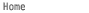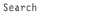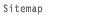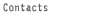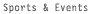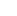© 2008 | Miami University | 501 East High Street | Oxford, Ohio 45056 | 513-529-1809 Equal opportunity in education and employment | Trouble viewing this page? accessibility@muohio.edu Privacy statement | Miami University is a smoke-free environment.